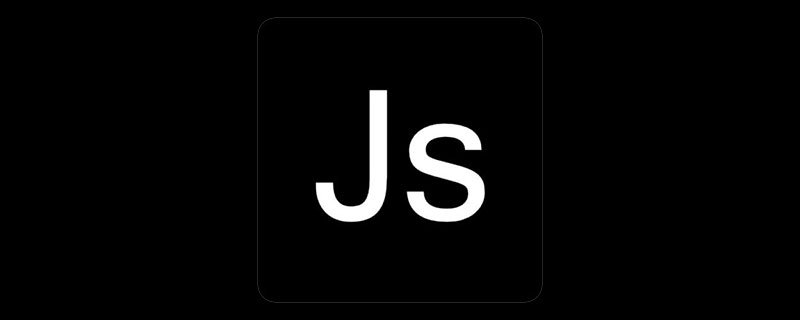# js保留两位小数的函数有哪些_亿码酷站_编程开发技术教程

【亿码酷站-编程开发教程】收集全网优质教程及源码资源！

js保留两位小数的函数有：1、【toFixed()】函数；2、【Math.floor()】函数不四舍五入 ，向下取整；3、使用字符串匹配方法；4、四舍五入保留2位小数；5、浮点数保留两位小数。js保留两位小数的函数有：

1、toFixed()方法

```// 1.四舍五入
var num =2.446242342;
num = num.toFixed(2);
console.log(num); //2.45
console.log(typeof num); // string```

2、Math.floor()，不四舍五入 ，向下取整

```// 2.不四舍五入 向下取整
num = Math.floor(num * 100) / 100;
console.log(num); //2.44
console.log(typeof num); // number```

3、字符串匹配

```// 3.不四舍五入 字符串匹配再转换
num = Number(num.toString().match(/^\d+(?:\.\d{0,2})?/));
console.log(num); //2.44
console.log(typeof num); // number```

4、四舍五入保留2位小数（若第二位小数为0，则保留一位小数）

```//4.四舍五入保留2位小数（若第二位小数为0，则保留一位小数）
function keepTwoDecimal(num) {
var result = parseFloat(num);
if (isNaN(result)) {
return false;
}
result = Math.round(num * 100) / 100;
return result;
};
keepTwoDecimal(num);
console.log(num); //2.44
console.log(typeof num); //number```

5、四舍五入保留2位小数（不够位数，则用0替补）

```//5.四舍五入保留2位小数（不够位数，则用0替补）
function keepTwoDecimalFull(num) {
var result = parseFloat(num);
if (isNaN(result)) {
return false;
}
result = Math.round(num * 100) / 100;
var s_x = result.toString(); //将数字转换为字符串
var pos_decimal = s_x.indexOf('.'); //小数点的索引值
// 当整数时，pos_decimal=-1 自动补0
if (pos_decimal < 0) {
pos_decimal = s_x.length;
s_x += '.';
}
// 当数字的长度< 小数点索引+2时，补0
while (s_x.length <= pos_decimal + 2) {
s_x += '0';
}
return s_x;
}
console.log(keepTwoDecimalFull(120.5)); //120.50
console.log(typeof keepTwoDecimalFull(120.5)); //string
console.log(keepTwoDecimalFull(2.446242342)); //2.45
console.log(typeof keepTwoDecimalFull(2.446242342)); //string```

1、将浮点数四舍五入，取小数点后2位

```//浮点数保留两位小数
//1.功能：将浮点数四舍五入，取小数点后2位
function toDecimal(x) {
var f = parseFloat(x);
if (isNaN(f)) {
return;
}
f = Math.round(x*100)/100;
return f;
}
console.log(toDecimal(3.1465926)); // 3.15
console.log(typeof toDecimal(3.1415926)); //number```

2、强制保留2位小数，如：2，会在2后面补上00.即2.00

```//2.强制保留2位小数，如：2，会在2后面补上00.即2.00
function toDecimal2(x) {
var f = parseFloat(x);
if (isNaN(f)) {
return false;
}
var f = Math.round(x*100)/100;
var s = f.toString();
var rs = s.indexOf('.');
if (rs < 0) {
rs = s.length;
s += '.';
}
while (s.length <= rs + 2) {
s += '0';
}
return s;
}
console.log(toDecimal2(3.1)); // 3.10
console.log(typeof toDecimal2(3.1415926)); //string```

3、保留两位小数 浮点数四舍五入 位数不够 不补0

```// 3.保留两位小数 浮点数四舍五入 位数不够 不补0
function fomatFloat(src,pos){
return Math.round(src*Math.pow(10, pos))/Math.pow(10, pos);
}
console.log(fomatFloat(3.12645,2)); // 3.13
console.log(typeof fomatFloat(3.1415926)); //numbe```

js保留两位小数的函数有哪些

mysql怎么修改列名为主键？

## Javascript如何使网页跳转

Javascript如何使网页跳转

## javascript中src是什么意思

javascript中src是什么意思